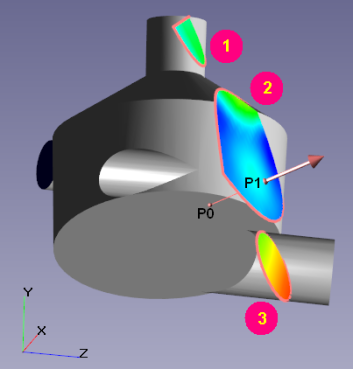## CHARACTERISTICS IN FLOWVISION: THE COMPLETE GUIDE - Characteristics applied to a surface

### DIFFERENCE IN CHARACTERISTICS CREATED ON A PLANE / SUPERGROUP

There is a big difference between Characteristics created on a Supergroup on a boundary condition, and those created on a plane or object surface, exactly replicating that Supergroup.

The variable values for Characteristics created on a Supergroup are recorded from the boundary condition. But for Characteristics built on a plane / object surface, values are recorded from the centers of the control cells at the boundary. These values are almost always different.

### CHARACTERISTIC ON A SELECTED CONTOURLet us consider a case when the plane on which a Characteristic is constructed passes through several calculation subregions or forms several closed contours. Then the question arises, what exactly does the characteristic calculate on such a plane?

If the plane intersects several calculated subareas, then when calculating the Characteristics on the plane, the subregion is selected in accordance with the position of the reference point.

If the plane intersects the geometry of the subregion in such a way that several closed contours are formed, then:

- by default, the characteristic is calculated across all contours
- you can select a single contour across which the characteristic will be calculated
To do this, place the reference point inside the contour of interest and select ‘integration along the selected contour’ in the Characteristic properties.

Other features of plotting Characteristics on a plane are described in the documentation.